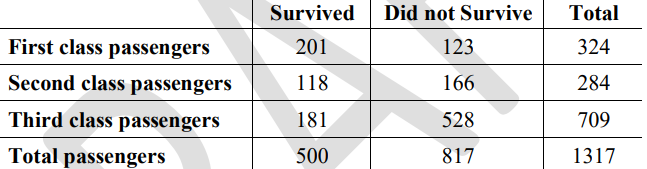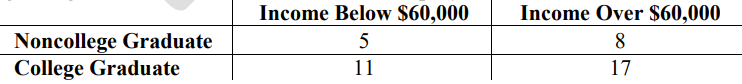# Standard #: MA.912.DP.4.4

This document was generated on CPALMS - www.cpalms.org

Interpret the independence of two events using conditional probability.

### General Information

Subject Area: Mathematics (B.E.S.T.)
Strand: Data Analysis and Probability
Status: State Board Approved

### Terms from the K-12 Glossary

• Conditional relative Frequency
• Event
• Experimental probability
• Frequency table
• Joint frequency
• Sample space
• Theoretical probability

### Vertical Alignment

Previous Benchmarks

Next Benchmarks

### Purpose and Instructional Strategies

In grade 7 and grade 8, students began working with theoretical probabilities and comparing them to experimental probability. In Mathematics for College Statistics, students determine if two events are independent of each other. Independence in this sense means that knowing whether one event occurred does not change the probability of the other event. For this benchmark, students determine independence using conditional probabilities. Two events, A and B, are independent if P(A | B) = P(A) and P(B | A) = P(B).
• Instructions includes using the product of probabilities to check independence (MA.912.DP.4.2). If P(A ∩ B) = P(A) x P(B), then the events are independent.
• Instruction distinguishes independence from mutually exclusive events in order to differentiate different types of joint probabilities.
• Instruction clarifies that mutually exclusive events (AB) = 0, which means the two events cannot occur at the same time.
• Instruction includes examples that demonstrate that P(A | B) ≠ P(B | A) unless P(A) = P(B)
• Instruction notes that when we check for independence in real world data sets, it is rare to get perfectly equal probabilities. We often assume that events are independent and test that assumption on sample data. If the probabilities are significantly different, then we conclude the events are not independent.
• Instruction includes the use of technology whether it be a statistical program or graphing calculators.
• Instruction includes the use of real-world data. In recent years there has been a push to move away from games of chance when discussing joint probabilities, conditional probabilities and independence in college statistics. The focus is more on contingency tables as this can begin to flow into hypothesis testing for proportions.

### Common Misconceptions or Errors

• Students may confuse what it means to be dependent and independent.
• Students may confuse independence with mutually exclusive events.
• Students may have difficulty recalling how to convert fractions, decimals and percentages.
• Students may think the symbol used for conditional probability is a slash that would be used to represent division and simply divide the probability of A by the probability of B.
• Students may get confused as to which event probability should be the denominator.
• Students may get confused when working with a two-way table that they need to restrict their answer to a certain section that is from the “given” conditional piece.
• For example, when given the condition of male they are only looking in the row or column containing males to get the total.
• Students who have difficulty with the terminology and notation will also have difficulty in understanding what is being asked by the questions..• Part A. Are the events “passenger survived” and the “passenger was in first class” independent events?
• Part B. Are the events “passenger survived” and the “passenger was in third class” independent events?
• Part C. Did all passengers aboard the Titanic have the same probability of surviving? Defend your response using calculations. Be prepared to share your reasoning..

### Instructional Items

Instructional Item 1
• The hiring department of Trinity United Mortgage Company compiled the accompanying data regarding the income and education of its employees.• Using conditional probabilities, are the events “employee is a college graduate” and the “employee makes over \$60,000” independent? Explain.

*The strategies, tasks and items included in the B1G-M are examples and should not be considered comprehensive.

#### Related Courses

 Course Number1111 Course Title222 1200340: Algebra 2 Honors (Specifically in versions: 2014 - 2015, 2015 - 2022, 2022 and beyond (current)) 1210300: Probability and Statistics Honors (Specifically in versions: 2014 - 2015, 2015 - 2019, 2019 - 2022, 2022 and beyond (current)) 7912070: Access Mathematics for Liberal Arts (Specifically in versions: 2014 - 2015, 2015 - 2018, 2018 - 2019, 2019 - 2022, 2022 - 2023, 2023 and beyond (current)) 1210305: Mathematics for College Statistics (Specifically in versions: 2022 and beyond (current)) 1207350: Mathematics for College Liberal Arts (Specifically in versions: 2022 and beyond (current))

#### Perspectives Video: Expert

 Name Description Let's Make a Math Deal Should I keep my choice or switch?  Learn more about the origins and probability behind the Monty Hall door picking dilemma and how Game Theory and strategy effect the probability. Download the CPALMS Perspectives video student note taking guide.
Printed On:10/2/2023 7:56:38 AM
Print Page | Close this window